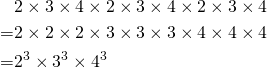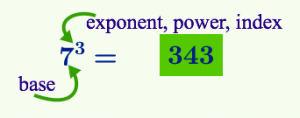# Exponents Introduction

Using an exponent is a short hand form of writing repeated multiplication or repeated division.

The idea of short hand forms is not new: multiplication is a short hand form of writing repeated addition. For example:Writingis much easier than writing out the long addition.

As with any new notation in mathematics, we need to know what it means before we can use it well.

Here is an example of repeated multiplication:There are eight twos on the left hand side. This is how we use exponent notation for this repeated multiplication:The other thing we know about multiplication is that we can multiply numbers in any order (commutativity property of real numbers).Now let’s use the commutativity property, and our new exponent notation to simplify this long expression:# A Sequence: Repeated Multiplication; Repeated Division

Move the slider to the right to multiply by 2.

Move the slider left to divide by 2.

Sequences always have a place to start (but not necessarily an end). The starting number here is, but when we multiply by 1 in math, we rarely write it. You can think for positive three “one multiply by 2 three times over”; and for negative three,  “one divide by 2 three times over”.

To learn about exponents, its useful to have some exponent facts memorized. The familiar values are square numbers, cube numbers, the first ten powers of 2 and the first 5 powers of 3.

Complete the table on Exponent Number Facts to Memorize to see how many exponent number facts you already have. The solution pdf is ENFM Solutions. Then test yourself with the following applet.

# Notation

There are three words used for an exponent, they are used interchangeably.# Why Exponents?

By learning about exponents we lay the foundation for several areas of learning. Here are four:

 Math Description Scientific Notation A way of measuring exceedingly small values (the weight of a fruit fly) and exceedingly large values (the speed of light) using familiar units (kg, meters etc). Growth and Decay Exponential functions allow us to mathematically model how populations (eg of people, of bats, of bacteria) grow; and to model how materials like uranium decay over time. Money Savings accounts and loans, left to themselves, increase exponentially. To understand how this works, we need to understand exponents. Prime Factors Finding prime factors is a little like finding out the ingredients in a good soup. Prime factors help us see how numbers are related to each other. For example,; and the number.

Number Sense Activity 1:

Compare the first five powers of 4 with the first ten powers of 2. Explain the pattern.  Choose various values ofandsuch that the following equation is true:Number Sense Activity 2:

Write three different equations, choosing values forandcarefully such that the equationis true. Check your equations with a calculator.